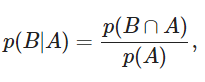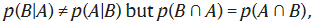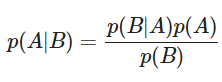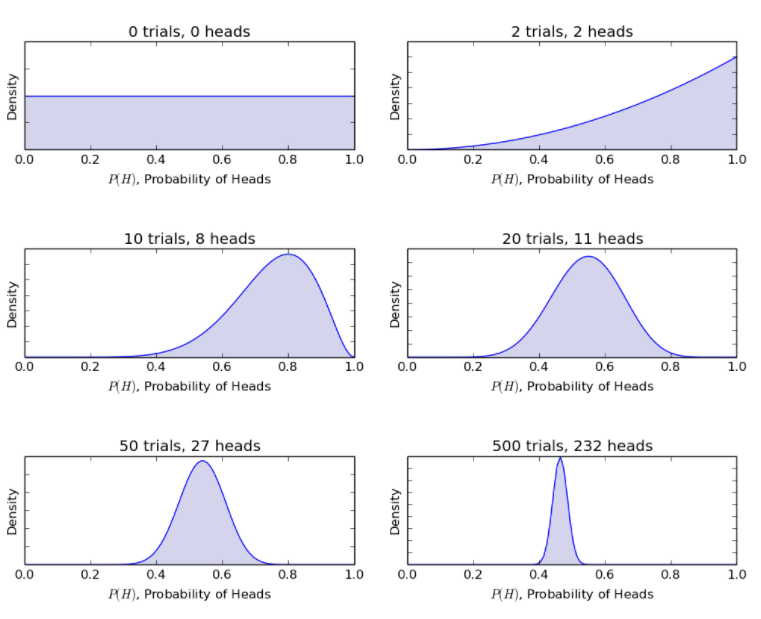HomeBlogArtificial Intelligences USBayesian Statistics and Model: Explained

# Bayesian Statistics and Model: Explained

Last updated:
29th Sep, 2021
Views
9 MinsView AllThe Bayesian technique is an approach in statistics used in data analysis and parameter estimation. This approach is based on the Bayes theorem.

Bayesian Statistics follows a unique principle wherein it helps determine the joint probability distribution for observed and unobserved parameters using a statistical model. The knowledge of statistics is essential to tackle analytical problems in this scenario.

Ever since the introduction of the Bayes theorem in the 1770s by Thomas Bayes, it has remained an indispensable tool in statistics. Bayesian models are a classic replacement for frequentist models as recent innovations in statistics have helped breach milestones in a wide range of industries, including medical research, understanding web searches, and processing natural languages (Natural Language Processing).

For example, Alzheimer’s is a disease known to pose a progressive risk as a person ages. However, with the help of the Bayes theorem, doctors can estimate the probability of a person having Alzheimer’s in the future. It also applies to cancer and other age-related illnesses that a person becomes vulnerable to in the later years of his life.

## Frequent Statistics Vs Bayesian Statistics

Frequent Statistics vs Bayesian statistics has consistently been a topic of controversy and nightmares for beginners, both of whom have difficulty choosing between the two. In the early 20th century, Bayesian statistics underwent its share of distrust and acceptance issues. With time, however, people realized the applicability of Bayesian models and the accurate solutions it yields.

Here’s taking a look at frequent statistics and the complexities associated with them:

### Frequent Statistics

It is a widely used inferential methodology in the world of statistics. It analyzes whether or not an event (mentioned as a hypothesis) has taken place. It also estimates the probability of the event occurring during the span of the experiment. The experiment is repeated until the desired outcome is achieved.

Their distribution samples are of actual size, and the experiment is repeated infinite times theoretically. Here’s an example showing how frequent statistics can be used to study the tossing of a coin.

• The possibility of getting a head on tossing the coin once is 0.5 (1/2).
• The number of heads denotes the actual number of leads obtained.
• The difference between the actual number of heads and the expected number of heads will increase as the number of tosses increases.

So here, the result depends on the number of times the experiment is repeated. It is a major drawback of frequent statistics.

Other flaws associated with its design and interpretation techniques became evident in the 20th century when the application of frequent statistics to numerical models was at its peak.

### Limitations of Frequent Statistics

The three major flaws of frequent statistics are listed below:

#### 1. Variable p Values

The values of p measured for a sample with a fixed size in an experiment with a defined endpoint change with any change in the endpoint and sample size. It results in two p values for a single data which is incorrect.

#### 2. Inconsistent Confidence Intervals

CI (Confidence Interval) solely depends on sample size. It makes the stopping potential irrelevant.

#### 3. Estimated Values of CI

Confidence intervals are not a probability distribution, and their values for a parameter are only an estimate and not actual values.

The above three reasons gave birth to the Bayesian approach that applies probabilities to statistical problems.

### Birth of Bayesian Statistics

Reverend Thomas Bayes first proposed the Bayesian approach to statistics in his essay written in 1763. This approach was published by Richard Price as a strategy in inverse probability to forecast future events based on the past.

The approach is based on the Bayes theorem that is explained below:

#### Bayes’ Theorem

Rényi’s axiom of probability examines conditional probabilities, where the possibilities of event A and Event B occurring are dependent or conditional. The basic conditional probability can be written as:The probability of Event B occurring depends on Event A.

The above equation is the foundation of the Bayes rule, a mathematical expression of the Bayes theorem that states:Here, ∩ denotes intersection.

The Bayes rule can be written as:The Bayes rule is the foundation of Bayesian statistics, where the available information on a particular parameter in a statistical model is compared and updated with collected data.

The background knowledge is represented as the prior distribution, which is then compared and studied with the observed or collected data as a likelihood function to find out the posterior distribution.

This posterior distribution is used to make predictions about future events.

Applications of the Bayesian approach depend on the following parameters:

1. Defining the prior and data model
2. Making relevant inferences
3. Scrutinizing and streamlining the models

## What are Bayesian Neural Networks?

Bayesian Neural Networks (BNNs) are networks you create when you extend standard networks using the statistical methodology and alter posterior inference to keep track of over-fitting. Since it is a Bayesian approach, there is a probability distribution associated with the parameters of the neural networks.

They are used to solve complex problems where there isn’t a free flow of data available. Bayesian neural networks help control the overfitting in domains such as molecular biology and medical diagnosis.

One can consider a whole distribution of answers to a question rather than just one possibility using Bayesian neural networks. They help you determine model selection/comparison and address problems that involve regularization.

Bayesian statistics offer mathematical tools to rationalize and update subjective knowledge concerning new data or scientific evidence. Unlike the frequent statistical approach, it functions based on the assumption that probabilities depend on the frequency of events repeating under the same conditions.

In short, the Bayesian technique is an extension of an individual’s assumption and opinion. The key aspect of the Bayesian model that makes it more efficient is its understanding that individuals differ in their opinions based on the kind of information they receive.

However, as new evidence and data come up, the individuals have a point of convergence, the Bayesian inference. This rational updating is the special feature of Bayesian statistics that makes it more effective on analytical problems.

Here, the probability of 0 is applied when there is no hope for an event occurring, and the probability of 1 is applied when it is sure that the event will occur. A probability between 0 and 1 gives room for other potential outcomes.

Bayes rule is now applied to achieve Bayesian inference to obtain a better inference from the model.

## How do you apply Bayes Rule to Obtain Bayesian Inference?

Consider the equation:

P(θ|D) = P(D|θ.)P(θ) / P(D)

P(θ) denotes the prior distribution,

P(θ|D) denotes the posterior belief,

P(D) represents the evidence,

P(D|θ) indicates the likelihood.

The main objective of Bayesian inference is to offer a rational and mathematically accurate method for blending the beliefs with evidence to obtain updated posterior beliefs. The posterior beliefs can be used as prior beliefs when new data gets generated. Thus, Bayesian inference helps to update beliefs continuously with the help of Bayes’ rule.

Considering the same coin flipping example, the Bayesian model updates the procedure from before to posterior beliefs with new coin flips. The Bayesian method gives the following probabilities.Source

Thus, the Bayesian model allows rationalising an uncertain scenario with restricted information to a more defined scenario with a considerable amount of data.

## Notable Differences between the Bayesian Model and the Frequentist Model

Frequent statistics

Bayesian statistics

The goal is considered as a point estimate, and CI

The goal is considered as a posterior distribution

The procedure starts from the observations

The process starts from the prior distribution

Whenever new observations are made, the frequentist approach re-computes the existing model.

Whenever new observations are made, the posterior distribution ( ideology/ hypothesis) is updated

Examples: Estimation of mean, t-test, and ANOVA.

Examples: Estimation of the posterior distribution of mean and overlap of high-density intervals.

• It provides an organic and simple means to blend pre-conceived information with a solid framework with scientific evidence. The past information about a parameter can be used to form a prior distribution for future investigation. The inferences adhere to the Bayes theorem.
• The inferences from a Bayesian model are logical and mathematically accurate and not crude assumptions. The accuracy remains constant irrespective of the size of the sample.
• Bayesian statistics follow the likelihood principle. When two different samples have a common likelihood function for a belief θ, all inferences about the belief should be similar. Classical statistical techniques do not follow the likelihood principle.
• The solutions from a Bayesian analysis can be easily interpreted.
• It offers a conducive platform for various models like hierarchical models and incomplete data issues. The computations of all parametric models can be virtually tracked with the help of other numerical techniques.

## Successful Applications of Bayesian Models Across History

Bayesian methods had a lot of successful applications during World War II. A few of them are listed below:

• A Russian statistician, Andrey Kolmogorov, successfully used Bayesian methods to improve the efficiency of Russian artillery.
• Bayesian models were used to break the codes of German U boats.
• A French-born American mathematician, Bernard Koopman, helped the allies identify the location of German U boats with the help of Bayesian models to intercept the radio transmissions.

If you’d like to learn more about Bayesian statistics, here’s upGrad’s Advanced Certification in Machine Learning and Cloud to understand the underlying concepts through real-life industry projects and case studies. The 12-month course is offered by IIT Madras and supports self-paced learning.

Reach out to us for further details.Blog Author
Director of Engineering @ upGrad. Motivated to leverage technology to solve problems. Seasoned leader for startups and fast moving orgs. Working on solving problems of scale and long term technology strategy.
Get Free Consultation

Select CourseSelectBy clicking 'Submit' you Agree to

#### Our Best Artificial Intelligence Course

1What is the Bayesian statistics model used for?

Bayesian statistical models are based on mathematical procedures and employ the concept of probability to solve statistical problems. They provide evidence for people to rely on new data and make forecasts based on model parameters.

2What is Bayesian Inference?

It is a useful technique in statistics wherein we rely on new data and information to update the probability for a hypothesis using the Bayes' theorem.

3Are Bayesian models unique?

Bayesian models are unique in that all the parameters in a statistical model, whether they are observed or unobserved, are assigned a joint probability distribution.

## Suggested Blogs